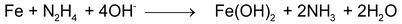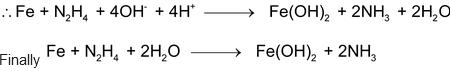# Ion Electron Method

## Ion Electron Method

### This method involves the following steps:

• Divide the complete equation into two half reactions, one representing oxidation and the other reduction.
• Balance the atoms in each half reaction separately according to the following steps:

(a) First of all balance the atoms other than H and O.

(b) In a reaction taking place in acidic or neutral medium, oxygen atoms are balanced by adding molecules of water to the side deficient in oxygen atoms while hydrogen atoms are balanced by adding H+ ions to the other side deficient in hydrogen atoms. On the other hand, in alkaline medium (OH-), for every excess of oxygen atom on one side is balanced by adding one H2O to the same side and 2OH- to the other side. In case hydrogen is still unbalanced, then balance by adding one OH-, for every excess of H atom on the same side and one H2O on the other side.

(c) Equalize the charge on both sides by adding suitable number of electrons to the side deficient in negative charge.

• Multiply the two half reactions by suitable integers so that the total number of electrons gained in one half reaction is equal to the number of electrons lost in the other half reaction.
• Add the two balanced half equations and cancel any term common to both sides.

There have been the common practices to balance the redox reaction by different methods like O.N. method and electron balance method. In the entrance examination it is never mentioned what method is to be used. We adopt here “quick” method that will certainly be a time-saving method.

(d) No other atom (except H and O) is unbalanced and therefore no need for this step.

(e)(f) Balance charge by H+Stoichiometry is related to the number of atoms or molecules of reacting species and product formed during the course of reaction which is governed by the law of chemical combination (discussed previously in Some Basic Concepts).

Related Topic

Here, we focus our attention about the stoichiometry related to redox reaction and acid and base neutralization reaction. As stoichiometric calculations are necessary for both analytical purpose (to find out chemical composition of given sample) and for assesing required amount of raw material to obtain desired mass of products.

For analytical purpose, most reactions held in liquid state, (i.e. aqueous solution) as solution provides better homogenity which helps in accurate measumenent with minimum error. This type of analysis in aqueous solution is known as volumetric anlalysis.